### Concept map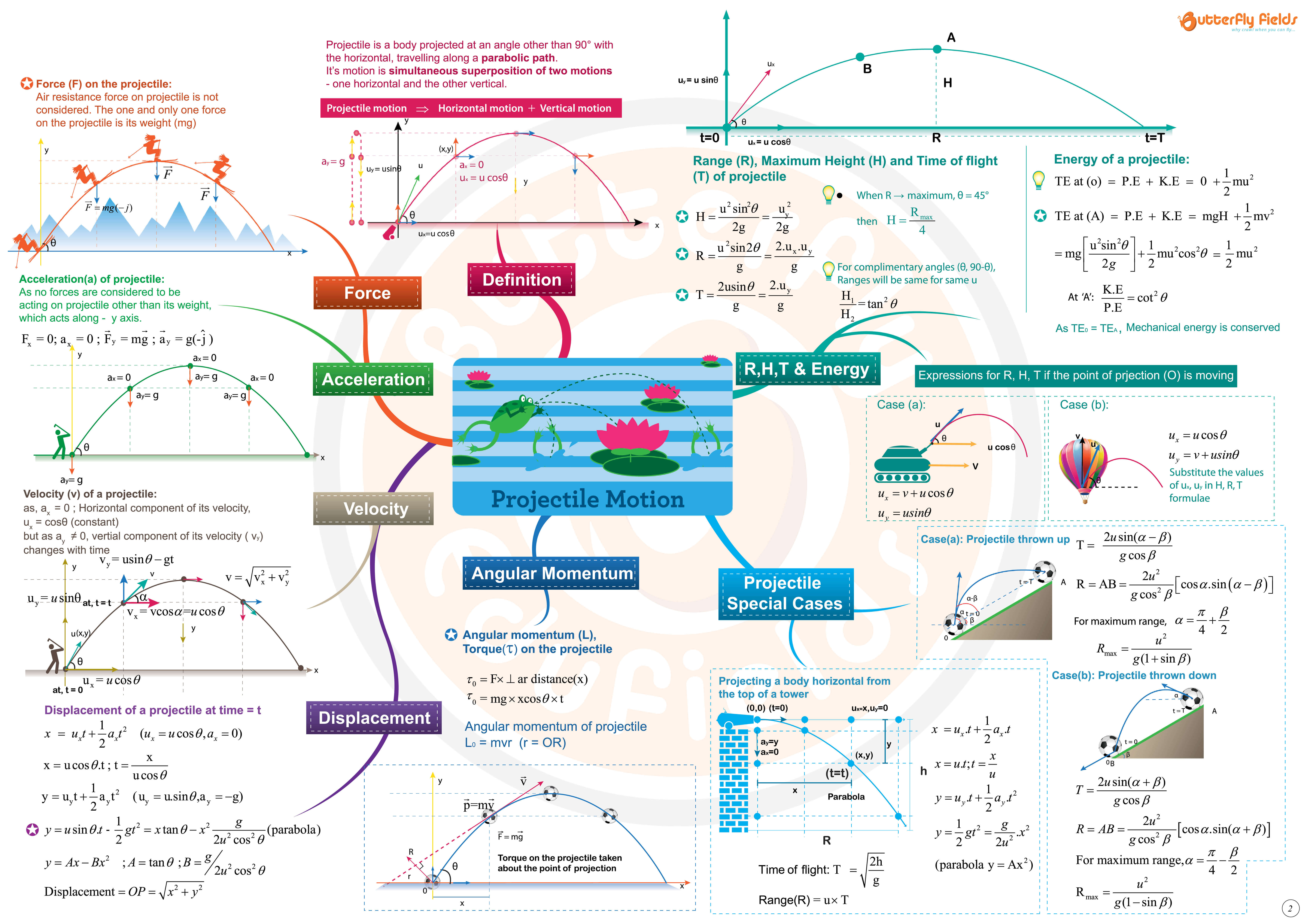A Concept map is a colorful, visual form of representing key concepts covered in a chapter . It can be used as an effective overview , learning and revision tool.

At its heart is a central topic of the chapter . This is then explored by means of branches representing main ideas, which all connect to this central idea.

Concept maps are metacognitive tools that give students awareness towards the process of learning and allow the representation of knowledge structures in thematic fields

### Questions

1. A projectile of mass 1 kg has two mutually perpendicular velocities 3m/s, 4 m/s at two different instances of time, during its path. What is its minimum kinetic energy?

2. A particle starts from origin of x,y coordinates at t = 0 and moves in x-y plane with a constant acceleration ‘α’ in y-direction. It’s equ- -ation of motion is, y =βx2. What is its velocity along x-direction ?

3. The equation of a projectile is y = px - qx2 . then R = ?

4) A projectile is projected at an angle 60° with the horizontal at 20 m/sec. At the instance, when its velocity makes an angle 30° with horizontal, the radius of curvature of its path is ------ (g = 10 m/s2)

5) If V1,V2 are the velocities at the end of a focal chord of the path of a particle and u is the horizontal component of velocity of projection, then u= ------ ?

6. A body is projected from the top of a tower of height ‘H’. The hori- -zontal velocity ‘V0’ , so that the body hits the inclined plane perpe- -ndicularly is ---- ?

7) A Projectile of mass(m) is projected with velocity ‘u’ at an angle (θ)with the horizontal. Find the change in its momentum in time = t is

8) A projectile is projected at an angle ‘θ’ with the horizontal with velo- -city ‘u’. After how much time, its velocity (v) is perpendicular to ‘u’. What is ‘v’ ?

9) Two balls are projected from the top and bottom of a tower so as to fall in a basket (B) on the horizontal ground. Which is at a distance 30√3 mt from the tower, as shown. Find the height of the tower

10) A particle is projected from the ground with an initial speed of ‘u’ at an angle ‘θ’ with horizontal, the average velocity of particle be- -tween its point of projection and highest point of trajectory is ......

11)The speed of a projectile at its maximum height is times its initial speed. If the range of the projectile is p times the maximum height attained by it,then p = -------

### A comprehensive revision tool for competitive exams

JEE (Mains), BITSAT, VITEE, KCET, MHCET, EAMCET, TANCET, MP PET, GUJCET, AIPMT, JIPMER, AFMC & Board Exams

“Easy way to interlink all key concepts without getting distracted by unnecessary details. Helps in quick review and fast revision.” - Arvind Saraf, JEE AIR 001

• Revise like never before!
• One topic in just one concept map
• Colored themes for grasp better understanding

### Shop Our Concept Map Books Collection### 40-in-1 DIY Robotics Master Kit | 8-15 yrs

Rs. 4,369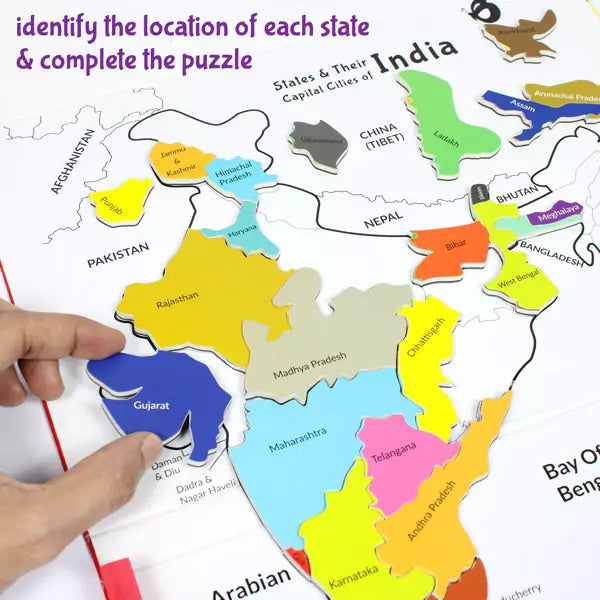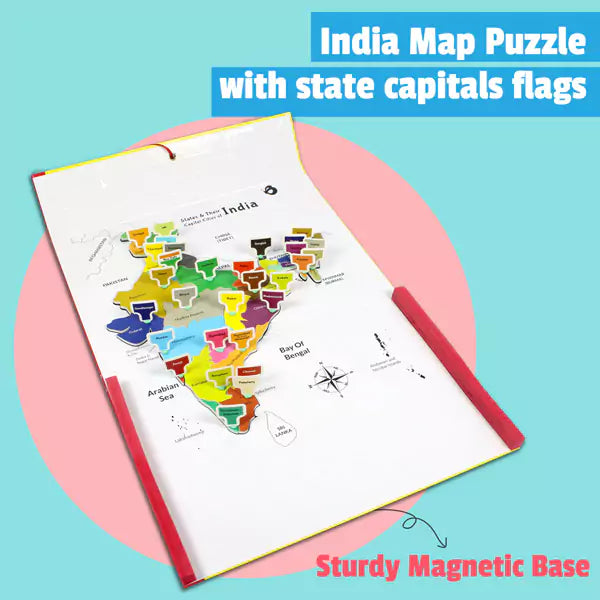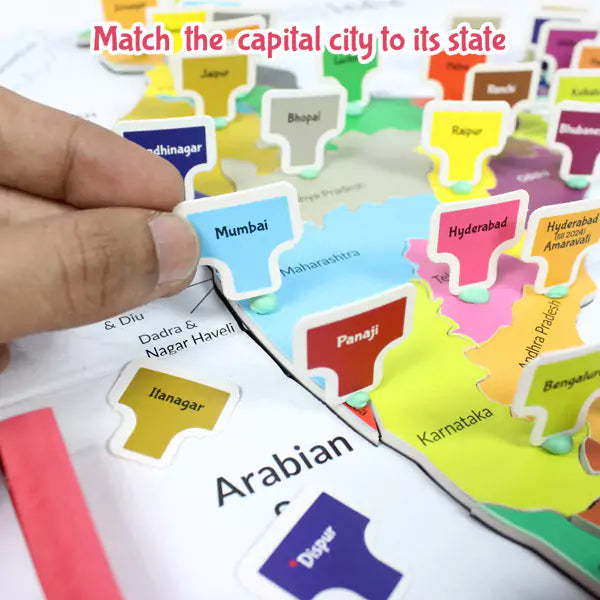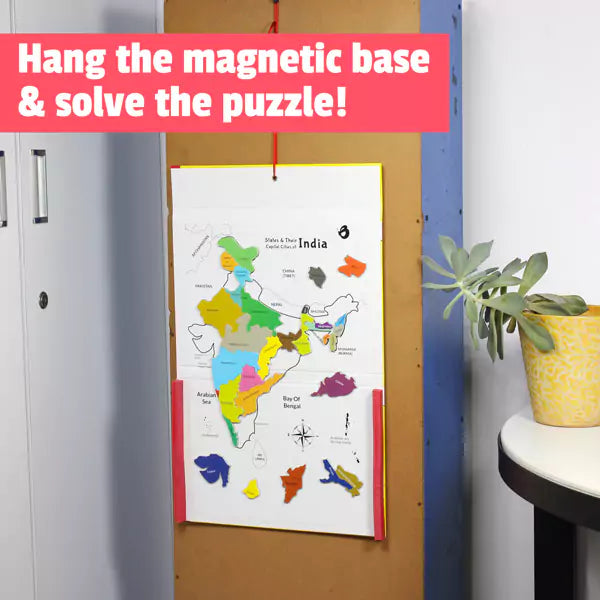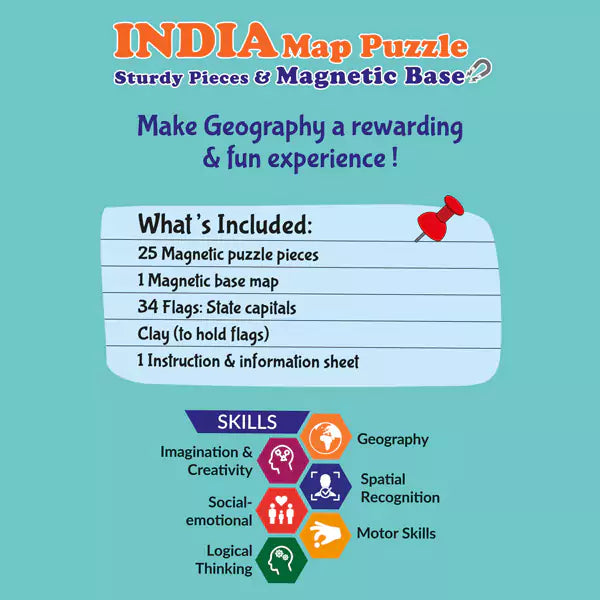### India Map Magnetic Puzzle | 6-8 yrs

Rs. 569### NEET, IIT JEE Physics + Chemistry Revision Guide 11 & 12 | 12+ yrs

Rs. 799Rs. 399

### Explore all topics

• Kinematics
• Projectile Motion
• Circular Motion
• Vectors
• Force
• Work Power Energy
• Centre Of Mass
• Law of Conservation of Momentum
• Gravitation
• Rotational Motion
• Simple Harmonic Motion
• Properties of matter and fluid mechanics
• Thermometry
• First Law of Thermo dynamics
• Second law of thermo dynamics
• Sound waves
• Ray optics
• Wave optics
• Electro Statics
• Current Electricity
• Thermal and Magnetic effects of Electric current
• EM Induction & AC circuits
• Atomic Physics
• Nuclear Physics
• EM Waves & Communication Devices
• Semi conductor devices
Sunday,Monday,Tuesday,Wednesday,Thursday,Friday,Saturday
January,February,March,April,May,June,July,August,September,October,November,December
Not enough items available. Only [max] left.
Shopping cart